﻿ How to Increase Voltage in a Circuit ﻿# How to Increase Voltage In a Circuit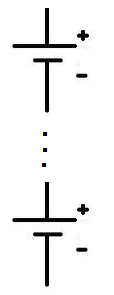In this article, we explain how to increase voltage in a circuit.

To increase voltage in a circuit, we place the individual voltages in series in a circuit.

### How to Increase DC Voltage

We'll begin with DC voltage.

To increase DC voltage in a circuit, we place the individual DC voltages in series in a circuit.

To connect voltages in series, we connect the negative polarity side of each of the voltage sources to the positiveHere you can see there are 3 DC voltage sources placed in series, since the negative side of each source connects to the positive side of the other source.

In this setup, the voltages add, so that:

Total Voltage= V1 + V2 + V3

### Examples

Let's we have a circuit below which provides 5 volts.We can increase this circuit voltage by adding another 5-volt power source in series with this voltage.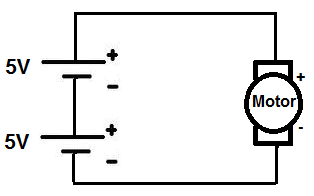Now the total voltage is 10 volts.

We can increase the circuit voltage to 15 volts by adding another 5-volt DC power source in series.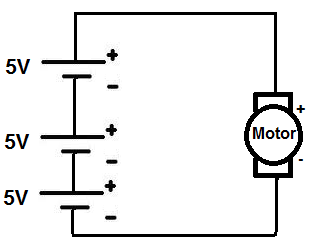Now the total voltage is 15V.

### How to Increase Voltage From Batteries

To increase voltage from batteries, we use the same concept as above, adding the batteries in series.

Let's start out with 1 AA battery in a circuit: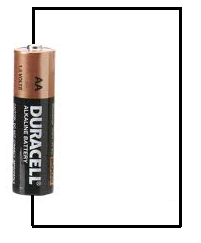1 single AA battery provides 1.5 volts.

Now if we add another battery in series to this battery, the voltages from both batteries add together and we get 3V of total voltage, since. 1.5 + 1.5= 3V.If we add yet another battery, the voltages from 3 batteries add together, giving us 4.5V, since 1.5V + 1.5V + 1.5V= 4.5V.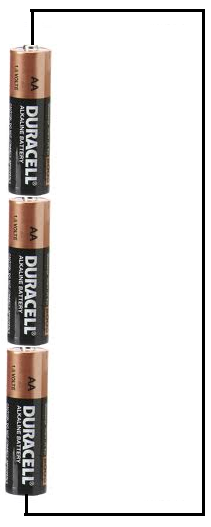And so on. We can keep increasing the voltage by adding more batteries in series.

### How to Increase AC Voltage

Increasing AC Voltage is the same as increasing DC voltage.

To increase the voltage, we connect the AC voltages in series to get a higher output voltage.

If the frequency of all the voltages are the same, the magnitude of the voltages simply add.

Look at the example below:The voltages will just add, so the total voltage will be 28Vac at 60Hz.

If the frequencies are different, then the voltages still add, but not directly. They must be converted to complex form and then added, because they are different frequenices.

Related Resources

HTML Comment Box is loading comments...

﻿